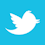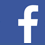Signal traces internal to the board change temperature along their length, changing resistance.

In our recent book,1 an image shows how heat from a relatively hot trace flows downward through the board (FIGURE 1). What is important to recognize here is how little horizontal heat dispersion there is. The heat seems to flow straight down. What thermal images like this obscure is the relative horizontal and vertical scales. The horizontal width in this image is 50mm, while the vertical height is less than 2mm. Not enough room is underneath the trace for much horizontal dispersion. Consequently, the temperature of the bottom layer of the board directly under the trace is only a few degrees cooler than the temperature of the top layer, regardless of what is beneath the top layer.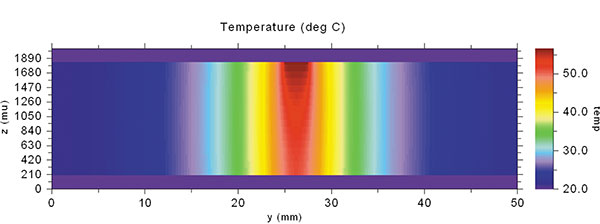Figure 1. Heat from a hot trace tends to flow straight down through a PCB.

We discuss this and its implications in some detail in another recent article2, but in this one, we want to suggest a different issue: whether this heat flow poses a potential signal integrity implication.

We will start with a simple model of a heated pad such as might be found under a BGA or microprocessor. We use a simulation tool called thermal risk management3 to do the analysis. We model a board that is 100mm (4")4 square with a 20mm (0.75"), 1oz. pad in the center of it. The board material is standard FR-4, 1.6mm (63 mils) thick. We apply enough power to raise its temperature to 62.9°C, 42.9° above the ambient. Under these conditions, the temperature directly underneath the pad on the bottom surface of the board is 61.2°C, 1.7° lower than the pad.

If we now place a 1oz. copper plane on the bottom of the board, the temperature of the pad will lower. Under these conditions, the temperature of the top pad is 40.1°C, and the temperature directly under the pad on the bottom layer plane is 35.6°C, a difference of 4.5°C.

FIGURE 2 illustrates the thermal gradients around the top layer of this model. The pad is not a uniform temperature. The center of the pad is the hottest at 40.1°. It cools least effectively. Heat conducts primarily down from the center. The corners of the pad cool most efficiently. The heat from there conducts down and out in a 90° arc. The sides of the pad are in between. They tend to cool down and straight out from the pad. As a result, the temperature drops off sharply to the sides of the pad, but the area directly under the pad stays fairly hot.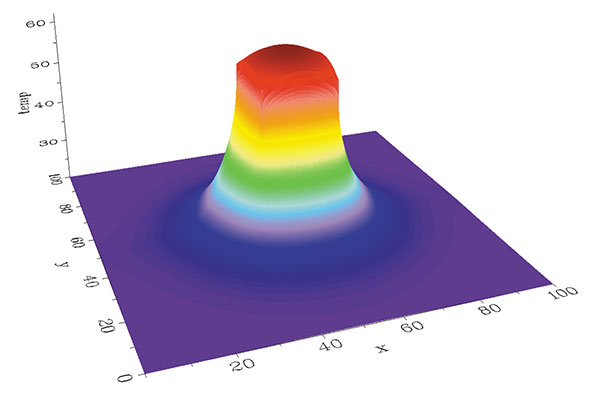Figure 2. Thermal gradients around the simulation.

If a trace is routed underneath the heated pad, assuming no self-heating, the temperature of the trace will have almost the same thermal profile as the pad. This is true whether the trace is below, above or originates underneath the heated pad, as in a BGA. We simulate this by routing a 90mm long (3.5") trace, 0.4mm (16 mil) wide, 1oz (0.033mm) thick on the middle layer of the board directly under the centerline of the pad at the point on the board where Y = 50mm.

FIGURE 3 illustrates the thermal profiles of our models along the X-axis (where Y = 50) of the top layer, middle layer and bottom layer of the board, with and without a plane on the bottom layer.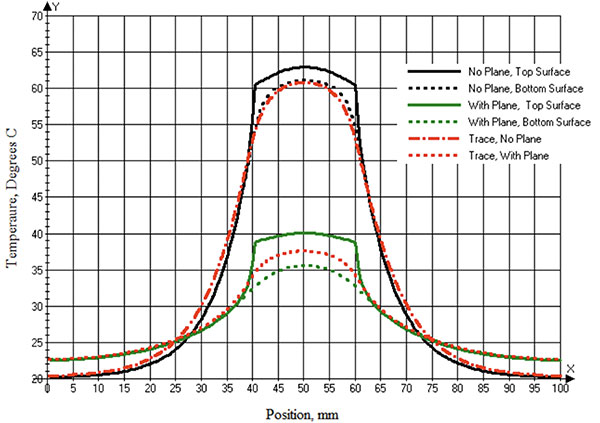Figure 3. Thermal profile of the top layer, bottom layer and trace layer of the simulation, with and without a bottom layer plane.

Elsewhere2, we postulated the bottom layer underneath a heated pad or trace would be less than 10° cooler than the heated pad or trace, regardless of what was underneath the pad or trace, in most practical situations. That is true in this simulation. There is only 1.8°C maximum difference between the top and bottom surfaces without a bottom layer plane and 4.5°C with a bottom layer plane. The maximum temperature of the trace is almost the same temperature as the bottom surface of the board in either case (shown here without a plane).

If there is a plane under a heated pad, the plane is within a few degrees of the pad. This has implications for thermal vias. A thermal via typically extends from a heated pad to an underlying copper surface, usually a plane. The formula for thermal conduction is: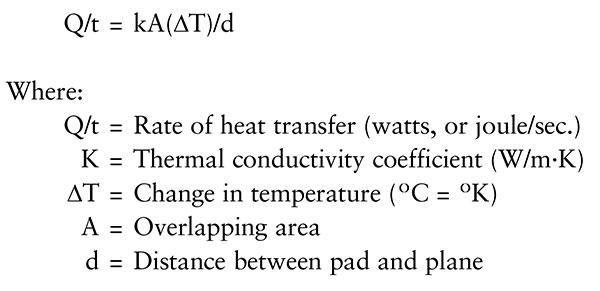EQ. 1

If the underlying surface is nearly the same temperature as the pad – for instance, ∆T is very small – then the thermal conductivity through the via is extremely low. This is why thermal vias are so inefficient cooling pads.5

If the trace changes temperature along its length, then it also must change resistance along its length because of the temperature coefficient of resistivity. TRM allows us to measure the resistivity at every point along the trace.6 If we know the resistivity and the trace dimensions, then we can calculate the point resistance at every point along the trace.7 FIGURE 4 graphs the point resistance along the trace simulated without a plane underneath it. The trace resistance under ambient conditions is 0.1149Ω. Under peak temperature conditions, the trace point resistance is 0.1332, a 15.9% increase. If we average the area under the curve, we calculate the average trace resistance is 0.1235Ω, using an ohmmeter.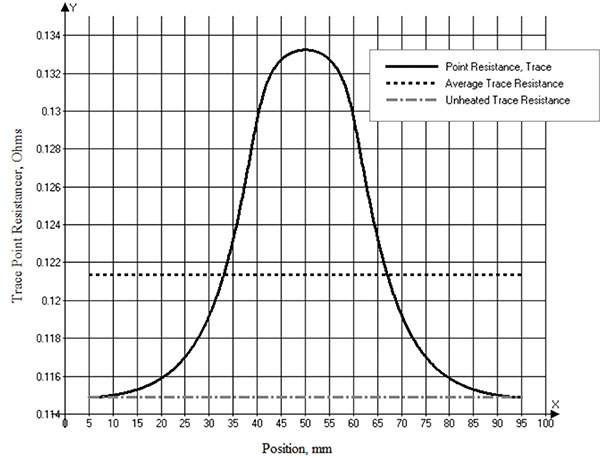Figure 4. Point and average resistance of the modeled trace under a heated pad.

We don’t need a thermal simulator to achieve a rough estimate of this effect. We can estimate the resistance of the trace under ambient conditions (20°C) by Equation 2.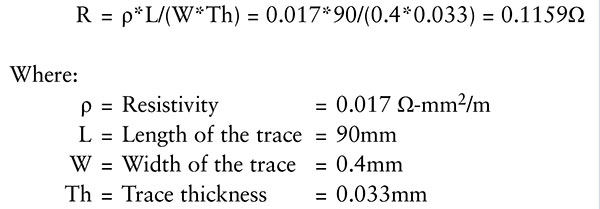EQ. 2

We can estimate the change in resistance by noting the temperature coefficient of resistivity is about 0.0038, and the change in temperature is about 42.9°C. Therefore, the point resistance at the maximum point is estimated by: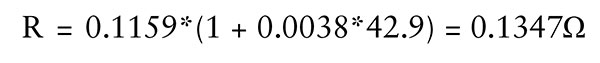EQ. 3

We can now estimate the average resistance of the entire trace by recognizing this new, elevated resistance occurs over 20mm of the total 90mm length, or 20/90 = 22.22% of the length. So: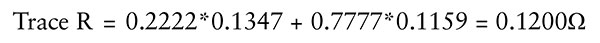EQ. 4

This approach overestimates the average resistance because it overestimates the temperature of the pad, ignoring the thermal gradients on the pad. It underestimates the total resistance because it underestimates the horizontal heat spreading at the edges of the pad. These two factors do not exactly cancel, but they do offset each other. The result is a rough estimate of what will happen when a trace crosses under – or over – a heated pad.

Now the question is: Could this change in resistance have signal integrity implications?

A. Suppose the trace is one side of a differential pair of traces. Would a difference in resistance of one side of the differential pair be enough to change the signal relationship along the trace and lead to a false signal?

B. Suppose we are dealing with rise times so quick or temperature changes great enough the characteristic impedance of the trace is nonlinear. Would the change in impedance be enough to cause a damaging signal reflection?

C. The changing resistance will lead to a changing voltage/current relationship. Could this cause a change in the electromagnetic field around the trace, resulting in an EMI or crosstalk issue?

These questions are beyond the scope of this paper, and we leave them to others to investigate. It is possible thermal changes like these are so small they are trivial, but someone should test that possibility.

We have based these questions on the results of a simulation. A relevant question is if we can replicate these results on a real board. We had a board available on which to test these results. It was small: 14.2 x 127mm x 1.6mm thick. It had a 4.5", 100-mil-wide trace on the top layer (the “victim trace”) with a 2.5mm (100 mil) trace crossing underneath it (the “aggressor trace”) on the bottom layer (FIGURE 5). We could apply current to the aggressor trace and measure both the temperature profile and the average resistance of the victim trace.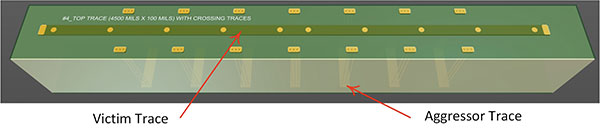Figure 5. Experimental trace available for testing.

There were difficulties defining all the parameters of the simulation model, however8:

1. Not all the relevant parameters were known about the board material, most important the thermal conductivity coefficients (in plane and through plane).
2. We were not certain of the resistivity of the copper traces.
3. We were not certain of the thickness of the copper traces, and in this type of investigation, the results are extremely sensitive to the trace thickness. A difference of 1µm in thickness makes a big difference in the results.
4. The numbers (trace resistance) we are dealing with here are extremely small, so comparisons are risky.

Nevertheless, we attempted to do so with acceptable results. FIGURE 6 illustrates the calculated victim trace temperature profile from the TRM model compared to the experimental result measured with a thermal imager. The two sets of observations are for an aggressor current of 8A and 11A. The maximum differences between the experimental results and the model results are less than 3°C (on the order of 5% or so), within the measurement error of this experimental setup. Note how much more sharply the curves peak in this case compared to Figure 3. That is because the heated trace is only 2.5mm wide compared to a 20mm square pad for the case above.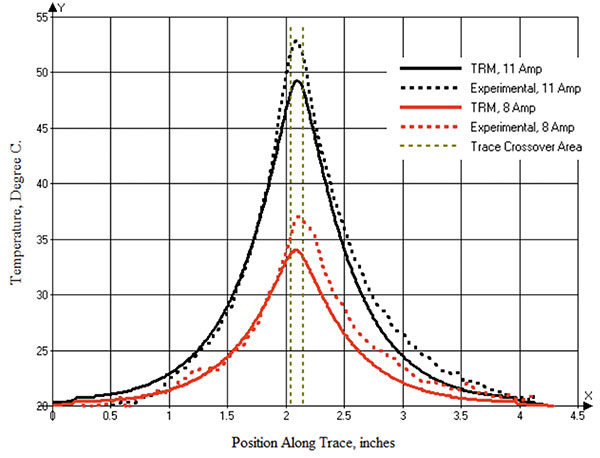Figure 6. Experimental temperature results vs. modeled results for the victim trace.

FIGURE 7 shows the simulated model resistance vs. the experimentally measured resistance for currents of 8A and 11A. The modeled resistances were determined the same way they were in Figure 4. We could only measure the trace (average) experimental resistance, but not the point resistance along the trace. As can be seen, the results are very close.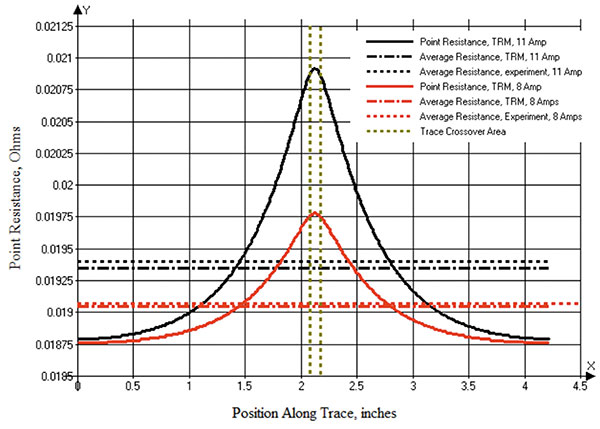Figure 7. Modeled vs. experimentally measured resistance along the victim trace.

The quantitative experimental results are reasonably close to the modeled results, but what is most encouraging is the shapes of the curves are almost exactly as expected.

We can conclude signal traces internal to the board can – and most assuredly do – change temperature along their length. Therefore, they change resistance. The question posed here is whether that change is significant enough to worry.

References

1.    Douglas Brooks and Johannes Adam, PCB Design Guide to Via and Trace Currents and Temperatures, https://uk.artechhouse.com/PCB-Design-Guide-to-Via-and-Trace-Currents-and-Temperatures-P2147.aspx, Artech House, February 2021. (See Chapter 7 and Figure 7.13.)
2.    Douglas Brooks and Johannes Adam, “Thermal management: A Close Look at Vertical Heat Flow in PCBs,” www.edn.com/thermal-management-a-close-look-at-vertical-heat-flows-in-pcbs/, EDN, Oct. 19, 2021.
3.    TRM was originally conceived and designed to analyze temperatures across a circuit board, taking into consideration the complete trace layout with optional joule heating, as well as various components and their own contributions to heat generation. TRM was written by Johannes Adam, Ph.D.
4.    Unit conversions are approximate.
5.    Douglas Brooks and Johannes Adam, “A Close Look at Facts and Myths About Thermal Vias,” www.edn.com/pcb-design-a-close-look-at-facts-and-myths-about-thermal-vias, EDN, Aug. 31, 2021.
6.    The resolution is determined by the thermal pixel setting. (See reference 1, Chapter 6.)
7.    Think of the “point resistance” as the resistance of the trace (in Ω) at that point. Resistance is a point concept because temperature, trace thickness, copper resistivity and dielectric thermal conductivity coefficients, among other things, are also point concepts. We define point resistance using the standard formula resistivity times length divided by conductor cross-sectional area – at a point. Total trace resistance is the average of all point resistances along the trace.
8.    We try to quantify uncertainties such as this in Chapter 7 of our book (Reference 1) and devote the entire Section 13.3 on the question of whether traces are uniform thickness. (They are not.)

Douglas Brooks, Ph.D., has bachelor’s and master’s degrees in electrical engineering from Stanford and a Ph.D. from the University of Washington. For the past 27 years, he has owned an engineering service firm and has published two books, including PCB Design Guide to Via and Trace Currents and Temperatures; This email address is being protected from spambots. You need JavaScript enabled to view it.. Johannes Adam, Ph.D., CID, has a doctorate in physics from University of Heidelberg. In 2009 he founded Adam Research and works as a technical consultant for electronics developing companies and as a software developer. Ulisses Castro has a bachelor’s in electrical engineering from Instituto Tecnológico de Tijuana and a master’s in engineering from Universidad Autónoma de Baja California. He has over 21 years of experience in manufacturing and electronic design.